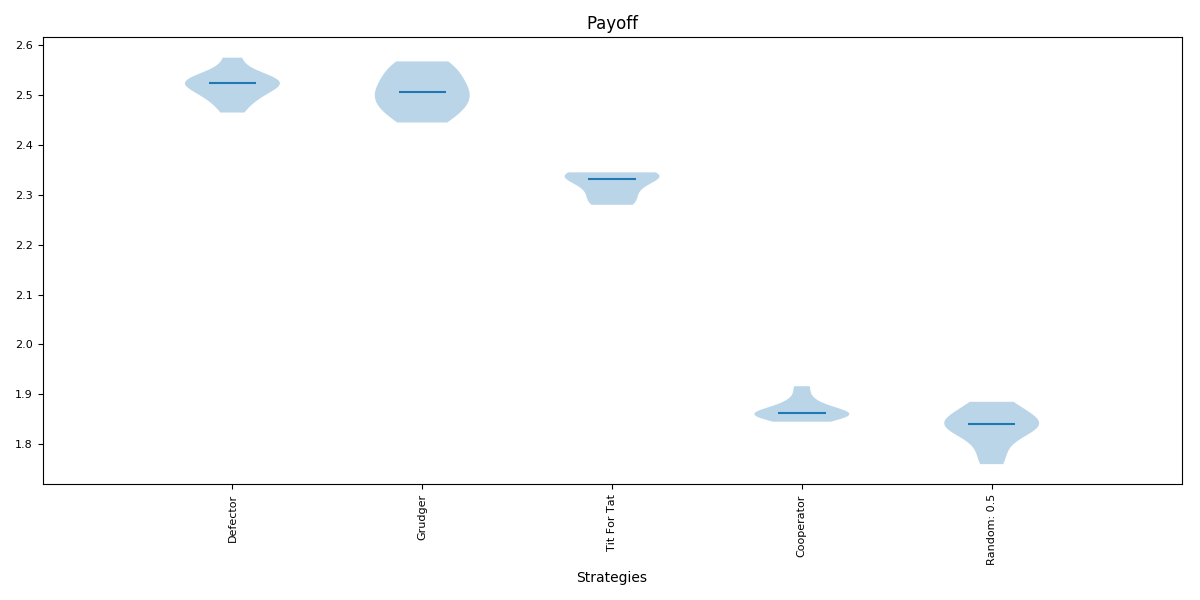# Visualising results¶

This tutorial will show you briefly how to visualise some basic results

## Visualising the results of the tournament¶

As shown in Creating and running a simple tournament, let us create a tournament, but this time we will include a player that acts randomly:

>>> import axelrod as axl
>>> players = [axl.Cooperator(), axl.Defector(),
...            axl.TitForTat(), axl.Grudger()]
>>> players.append(axl.Random())
>>> tournament = axl.Tournament(players)
>>> results = tournament.play()


We can view these results (which helps visualise the stochastic effects):

>>> plot = axl.Plot(results)
>>> p = plot.boxplot()
>>> p.show()## Visualising the distributions of wins¶

We can view the distributions of wins for each strategy:

>>> p = plot.winplot()
>>> p.show()## Visualising the payoff matrix¶

We can also easily view the payoff matrix described in Access tournament results, this becomes particularly useful when viewing the outputs of tournaments with a large number of strategies:

>>> p = plot.payoff()
>>> p.show()## Saving all plots¶

The axelrod.Plot class has a method: save_all_plots that will save all the above plots to file.

## Passing various objects to plot¶

The library give access to underlying matplotlib axes objects of each plot, thus the user can easily modify various aspects of a plot:

>>> import matplotlib.pyplot as plt
>>> _, ax = plt.subplots()
>>> title = ax.set_title('Payoff')
>>> xlabel = ax.set_xlabel('Strategies')
>>> p = plot.boxplot(ax=ax)
>>> p.show()